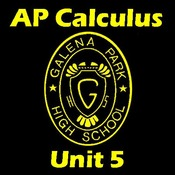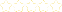• > Miguel Ramirez
• > AP Calculus Unit 5. Logarithmic and Exponential Functions
+# AP Calculus Unit 5. Logarithmic and Exponential Functions

Owner: Miguel Ramirez
##### Description:
Tutorial for Chapter 5 Larson-Hostetler-Edwards: Calculus of a Single Variable Galena Park High

### Your Quiz Points in this Playlist

Total Possible
0
11 Tutorials in this Playlist

#### 5.0 - Class Notes and Assignments

Author: Miguel Ramirez
Rating:(0)

#### 5.1.1 - The Natural Logarithmic Function. Differentiation

Author: Miguel Ramirez
Rating:(0)
This tutorial has a quiz
•• -

#### 5.1.2 - Logarithmic Differentiation

Author: Miguel Ramirez
Rating:(0)
This tutorial has a quiz
•• -

#### 5.2 - The Natural Logarithmic Function. Integration

Author: Miguel Ramirez
Rating:(1)
This tutorial has a quiz
•• -

#### 5.3 - Inverse Functions

Author: Miguel Ramirez
Rating:(0)
This tutorial has a quiz
•• -

#### 5.4.1 - Natural Exponential Function. Differentiation

Author: Miguel Ramirez
Rating:(1)
This tutorial has a quiz
•• -

#### 5.4.2 - Natural Exponential Function. Integration

Author: Miguel Ramirez
Rating:(0)
This tutorial has a quiz
•• -

#### 5.5.1 - Logarithms and Exponentials. Other Bases that e. Differentiation

Author: Miguel Ramirez
Rating:(0)
This tutorial has a quiz
•• -

#### 5.5.2 - Logarithms and Exponentials. Other bases that e. Integration

Author: Miguel Ramirez
Rating:(0)
This tutorial has a quiz
•• -

#### 5.6 - Inverse Trigonometric Functions. Differentiation

Author: Miguel Ramirez
Rating:(1)
This tutorial has a quiz
•• -